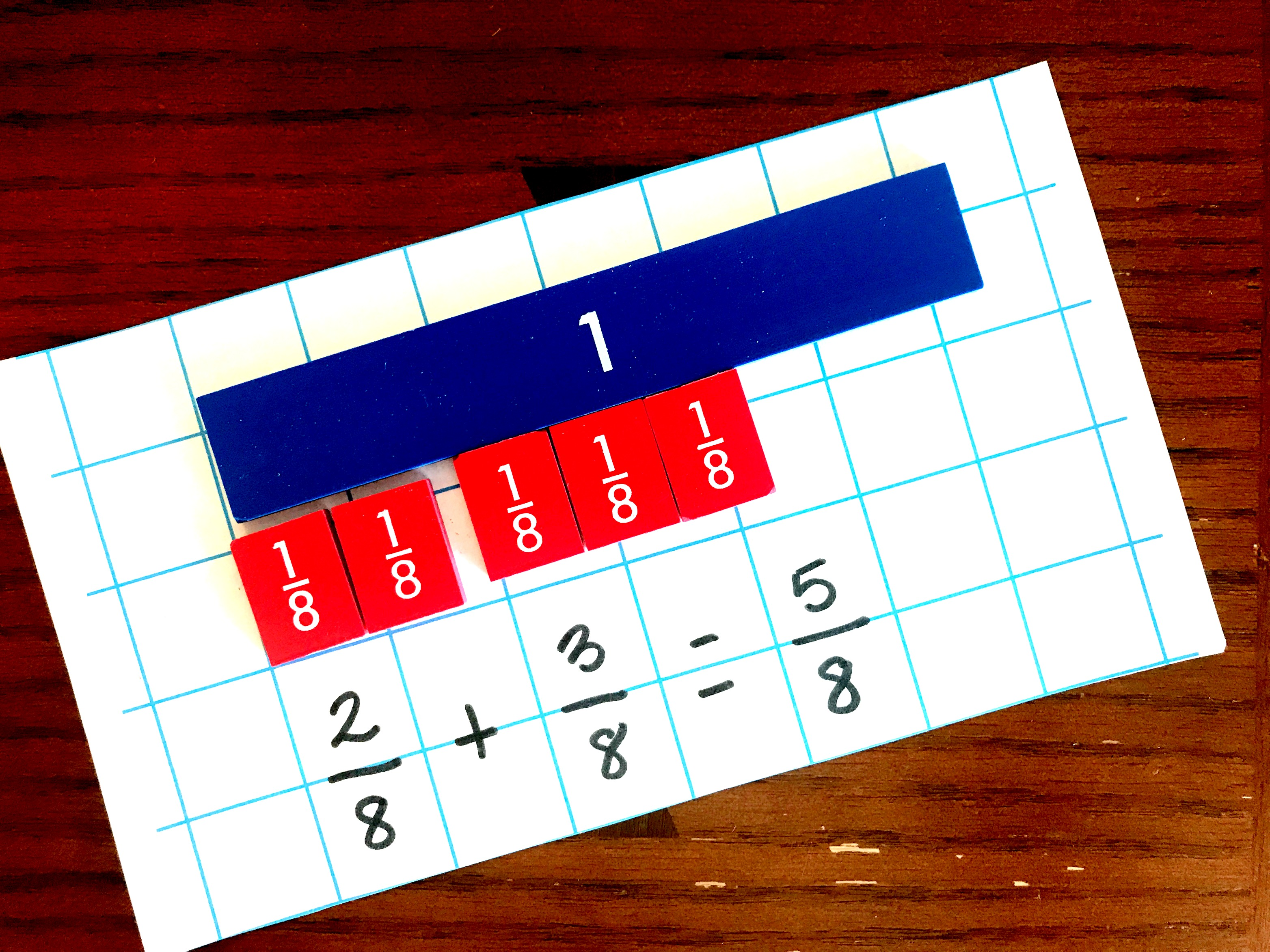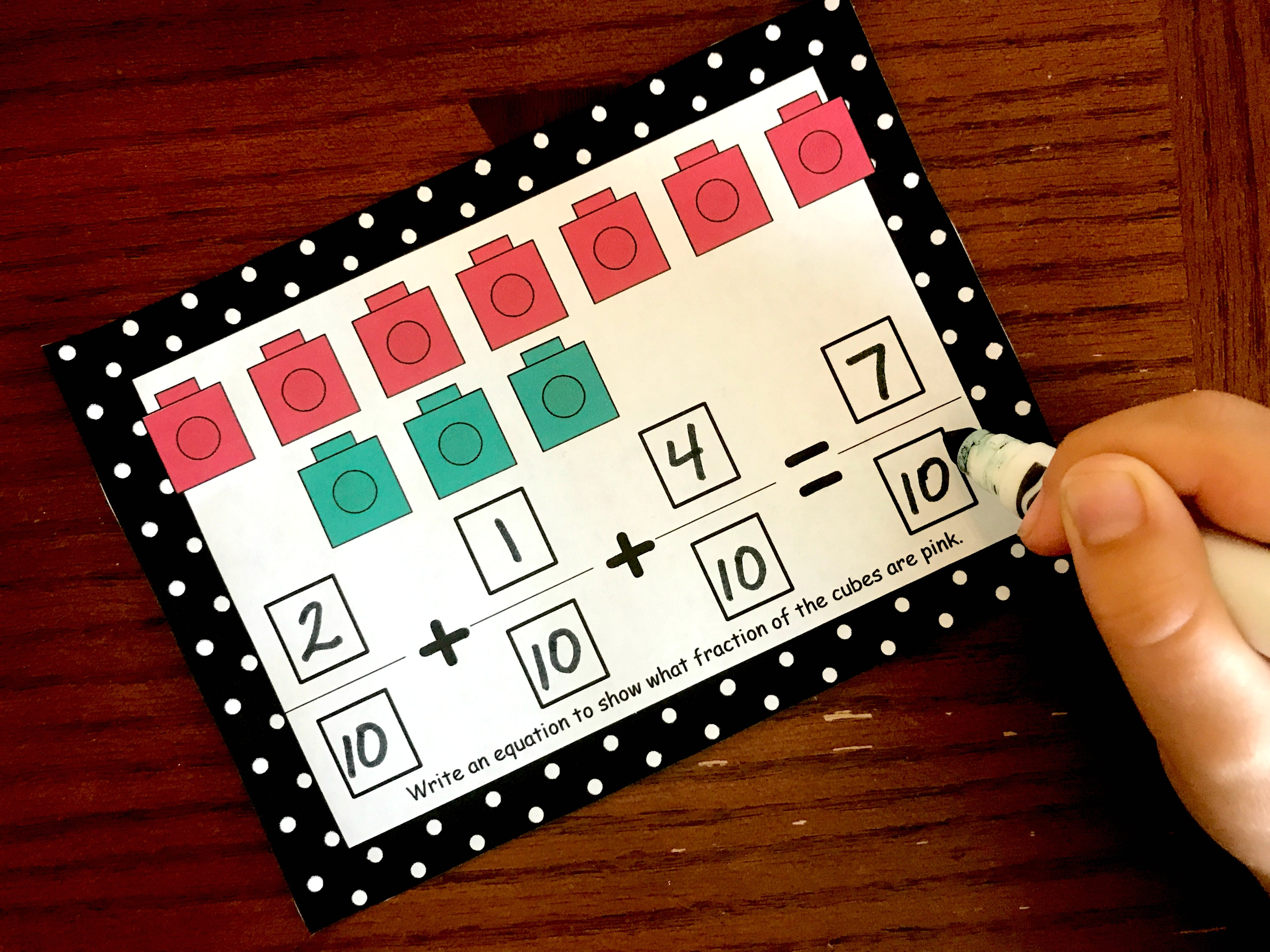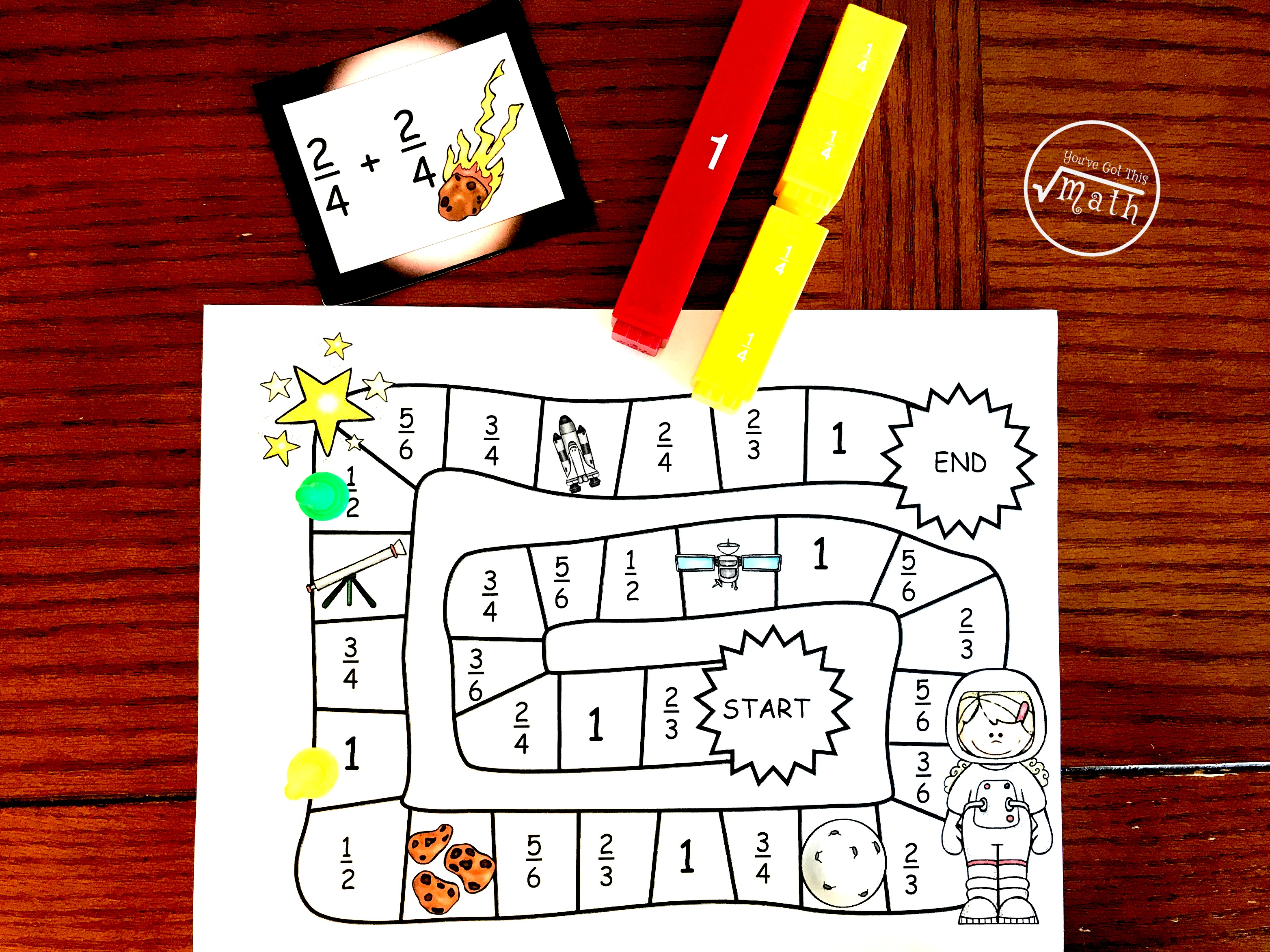Home » Math » Three Manipultives To Use When Teaching Decomposing Fractions

Three Manipultives To Use When Teaching Decomposing Fractions

Decomposing fractions is a wonderful way to develop fraction sense. Try using these three manipulatives to make it concrete. (Free Printable Included)The free printable can be found at the END of this post. Click on the blue “get your free printable here” button.

All month, we have discussed how to Develop Fraction Sense, and today I have another fantastic way to help children build an understanding of fractions.

Decomposing Fractions!!

I was first “introduced” to this concept when I was a fourth and fifth-grade math coach. We were faced with the daunting task of teaching new standards, and over and over we saw the words decompose a fraction. And so we tackled it head on, and we taught our students to decompose fractions.

What did we learn?

We learned that when children can decompose fractions, they are beginning to understand what fractions are! You can’t decompose a fraction unless you understand what a fraction represents.

So what is decomposing fractions? TutaPoint says, “To decompose a fraction means to find the two (or more) fractions that when added together give you the fraction with which you started.”

And once children learn to do it, they can conquer even more fractional concepts.

Decomposing Fractions with Pattern Blocks or Fraction CirclesPattern blocks are my go to for teaching fractions, and teaching decomposing fractions is no different. And if children are familiar with using pattern blocks for representing fractions, this is an easy transition.

1. First, have the children use pattern blocks to represent a fraction…in my example, I used 2/3.
2. Next, have the children show you the parts that equal 2/3….the two rhombi.
3. Finally, have them create an equation that represents the pattern blocks in front of them….1/3 + 1/3 = 2/3

You can also do this activity with fraction circles.

Decomposing Fractions with Fraction BarsNext, try out fraction bars. These may be easier than pattern blocks as the fractions are written directly on them. Once again, give the children a fraction to represent, have them break that fraction a part, and then create an equation.

In this example, the child is representing five-eighths. Next, they broke apart the representation into 2/8 and 3/8. Finally, they created the expression 2/8 + 3/8 = 5/8

Decomposing Fractions With Snap CubesFor our last manipulative, I created a printable to help you!

Many times our fraction practice focuses on finding the fraction of a whole, but it is also important that children can find the fraction of a set or group of objects. Using snap cubes can help with that.

In the example above. seven-tenths of the cubes are pink and three-tenths are turquoise. The task card is asking the child to decompose the fraction that represents the pink cubes. They did that by creating the equation 2/10 + 1/10 + 4/10 = 7/10.The second set of task cards changes things around a little bit. They provide an expression, and the children have to create a representation of it. In this example, the child recognized that 5/11 + 3/11 + 1/11 = 9/11. They used 9 cubes to represent the numerator and then used 2 blue cubes so that there would be a total of eleven cubes. They were then able to solve the expressions and write in 9/11.

Enjoy playing with the manipulatives and using the task cards to challenge your kiddos.

You’ve Got This

Rachel

You may also be interested in:And be sure to check out the rest of our series on Developing Fraction Sense: